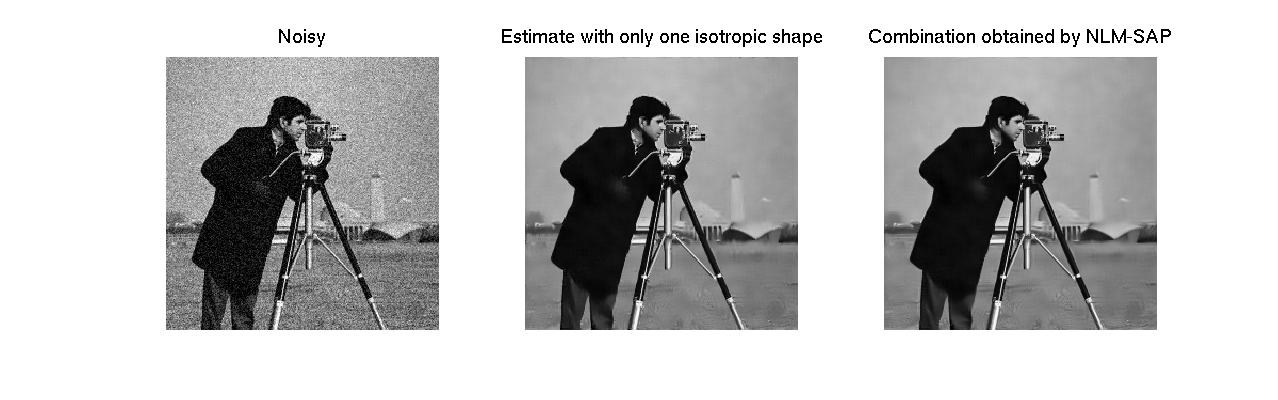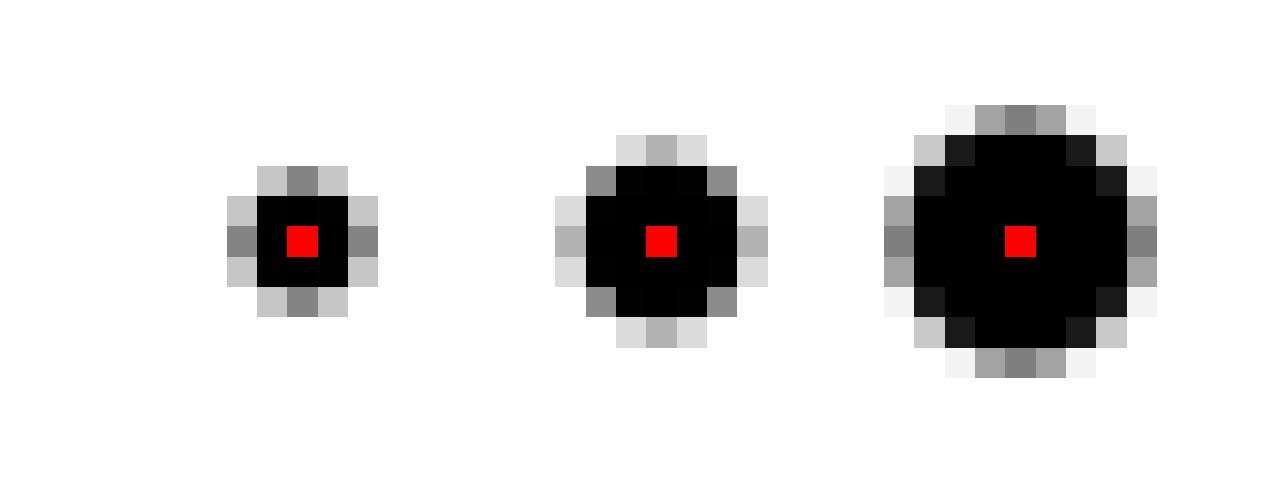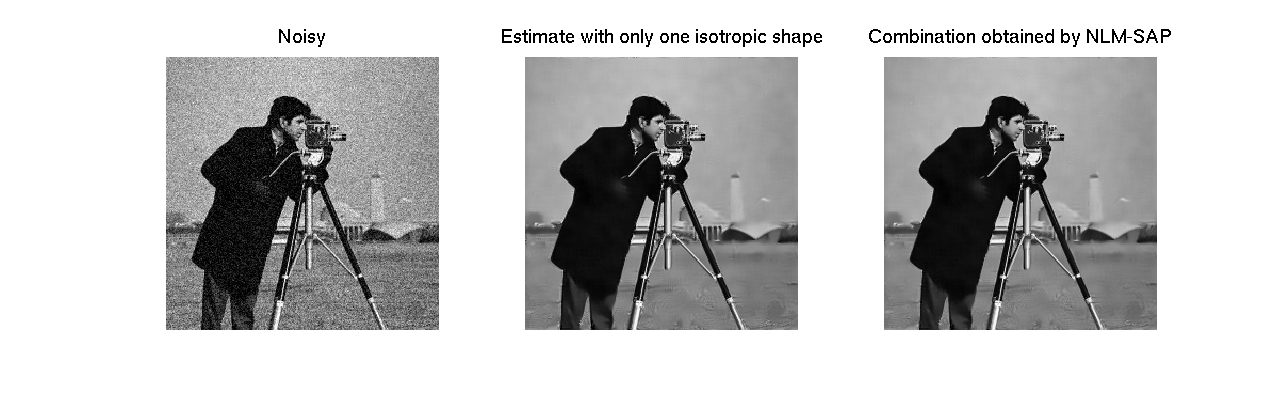# NLM Shape-Adapative Patches (NLM-SAP)

## Simple example on Cameraman

We present here the standard version of our NLM-SAP algorithm. The kernel used to compare the shapes is trapezoidal. See also README.TXT in the associate zip file for more details.

First demo (DEMO_NLMSAP.m): We use 4 directionnal quarter pies and an isotropic one, all of them with 3 scales (15 shapes in total). We illustrate the benefits of combining the estimates based on different shapes rather than using only a single one.

Second demo (DEMO_NLMSAP_FAST.m): We use 3 isotropic Gaussian shapes. We illustrate the benefits of combining the estimates based on different sizes rather than using only a single one.

See DEMO_NLMSAP.m, DEMO_NLMSAP_FAST.m, DEMO_NLMSAP_GAUSS_8DIRECTIONS.m

close all
clear all
addpath('tools');
addpath('functions');


## Parameters initialization

sig             = 20;            % standard-deviation of the noise
hW              = 5;             % half-size of the search window
alpha           = 0.7;           % h^2=alpha^2*sigma^2/2 for 7x7 patches
% h^2 is adapted for other shapes in
% proportion of the 0.99-quantile.
temperature     = 0.4*sig.^2;    % Temperature for EWA


## Build the true and noisy images

img     = double(imread('cameraman.png'));
[M,N]   = size(img);


## Initialize the random seed

randn('seed', 2);
img_nse = img + sig * randn(size(img));


## Build the shapes

L = 2;           % number of size levels
R = 4;           % radius of the first level (evolution as R*sqrt(2)^(n-1)
A = 0;           % number of angle partitions (precisely 2^A)
shapes = cat(3, ...
build_pie(M,N,L,R/2,A), ...
build_pie(M,N,L,R/sqrt(2),A), ...
build_pie(M,N,L,R,A));


## Display the shapes

figure
plot_shapes(shapes);## Compute the NL-Shapes estimations

tic
[nlsum, sumphi, sumphi2, deriv] = ...
NLMSAP_trapezoid(img_nse, hW, shapes, alpha, sig);
nbshapes = size(nlsum, 3);

Apply NLM-SAP (Trapezoidal kernel) -- shape #1
Apply NLM-SAP (Trapezoidal kernel) -- shape #2
Apply NLM-SAP (Trapezoidal kernel) -- shape #3
Apply NLM-SAP (Trapezoidal kernel) -- shape #4
Apply NLM-SAP (Trapezoidal kernel) -- shape #5
Apply NLM-SAP (Trapezoidal kernel) -- shape #6
Apply NLM-SAP (Trapezoidal kernel) -- shape #7
Apply NLM-SAP (Trapezoidal kernel) -- shape #8
Apply NLM-SAP (Trapezoidal kernel) -- shape #9
Apply NLM-SAP (Trapezoidal kernel) -- shape #10
Apply NLM-SAP (Trapezoidal kernel) -- shape #11
Apply NLM-SAP (Trapezoidal kernel) -- shape #12
Apply NLM-SAP (Trapezoidal kernel) -- shape #13
Apply NLM-SAP (Trapezoidal kernel) -- shape #14
Apply NLM-SAP (Trapezoidal kernel) -- shape #15


## Compute risks maps

riskmat = risk_sure(img_nse, nlsum, deriv, sig);


## Filter risks maps

riskemp = (repmat(img_nse, [1 1 nbshapes]) - nlsum).^2;
divmat = riskmat - riskemp;
[divmat_diff riskmat_diff] = riskfilter_yaroslavsky(divmat, riskmat);


## Aggregation step with EWA

[img_NLMSAP, beta_NLMSAP] = ...
aggregation_EWA(nlsum, divmat_diff, temperature);


## Total time

toc

Elapsed time is 30.423400 seconds.


## Display result

figure('Position',[100 100  1400 400])

subplot(1,3,1)
plotimage(img_nse);
title('Noisy');
set(get(gca,'Title'),'FontSize',16);
subplot(1,3,2);
plotimage(nlsum(:,:,2));
title('Estimate with only one isotropic shape');
set(get(gca,'Title'),'FontSize',16);
subplot(1,3,3);
plotimage(img_NLMSAP);
title('Combination obtained by NLM-SAP');
set(get(gca,'Title'),'FontSize',16);
linkaxes## Build 3 disk shapes

L = 1;           % number of size levels
R = 4;           % radius of the first level (evolution as R*sqrt(2)^(n-1)
A = 0;           % number of angle partitions (precisely 2^A)
shapes = cat(3, ...
build_pie(M,N,L,R/2,A), ...
build_pie(M,N,L,R/sqrt(2),A), ...
build_pie(M,N,L,R,A));


## Display the shapes

figure('Position',[400 400  1500 500])
plot_shapes(shapes);## Compute the 3 NL-Shapes estimations

tic
[nlsum, sumphi, sumphi2, deriv] = ...
NLMSAP_trapezoid(img_nse, hW, shapes, alpha, sig);
nbshapes = size(nlsum, 3);

Apply NLM-SAP (Trapezoidal kernel) -- shape #1
Apply NLM-SAP (Trapezoidal kernel) -- shape #2
Apply NLM-SAP (Trapezoidal kernel) -- shape #3


## Compute risks maps

riskmat = risk_var(sumphi, sumphi2);


## Aggregation step with WAV

[img_fast_NLMSAP, beta_fast_NLMSAP] = aggregation_WAV(nlsum, riskmat);


## Total time

toc

Elapsed time is 6.153982 seconds.


## Display result

figure('Position',[100 100  1400 400])

subplot(1,3,1)
plotimage(img_nse);
title('Noisy');
set(get(gca,'Title'),'FontSize',16);
subplot(1,3,2);
plotimage(nlsum(:,:,2));
title('Estimate with only one isotropic shape');
set(get(gca,'Title'),'FontSize',16);
subplot(1,3,3);
plotimage(img_fast_NLMSAP);
title('Combination obtained by NLM-SAP');
set(get(gca,'Title'),'FontSize',16);
linkaxes"Anisotropic Non-Local Means with Spatially Adaptive Patch Shapes"
C.-A. J. Salmon, V. , SSVM 2011, PDF.

"Non-Local Methods with Shape-Adaptive Patches (NLM-SAP)"
C.-A. J. Salmon, V. , J. Math. Imaging Vis., vol.43, pp. 103-120, 2012, PDF.

Corresponding Matlab toolbox ZIP.

### Contact us

Please contact us if you have any question.

Comments are closed.